Courses
Courses for Kids
Free study material
Free LIVE classes
More

# Understanding Angle BisectorsLIVE
Join Vedantu’s FREE Mastercalss

## Introduction

Angle bisectors can be seen quite often in our daily life. They are defined as a ray or a line that splits an angle into two equal halves. It is important to know their use and methods to construct them.

## What Are Angle Bisectors and Their Use

Before we learn what angle bisectors are, Let’s look at what an angle is and what bisectors are. An angle is a combination of two rays having a common endpoint. This common endpoint is known as the vertex of the angle and the spacing between the rays is called the measure of an angle. A bisector is simply a line (a ray in our case) that divides the angle into two equal parts.Scissor Showing an Angle

The scissor pivot is called the angle's vertex. The line passing through the vertex divides the scissor into two symmetrical portions and is called the bisector. If a bisector bisects an angle, it is called an angle bisector.

Real-world applications for angle bisectors include quilting, baking, and architecture. Numerous games, including rugby, football, and pool, use angle bisectors.

## How to Draw an Angle Bisector?

Let’s take a fun approach in trying to make an angle bisector. Take a paper and make an angle on it. Now fold the paper so that the first line completely overlaps the second line. Make a crease. Now unfold the paper and draw a line along the crease.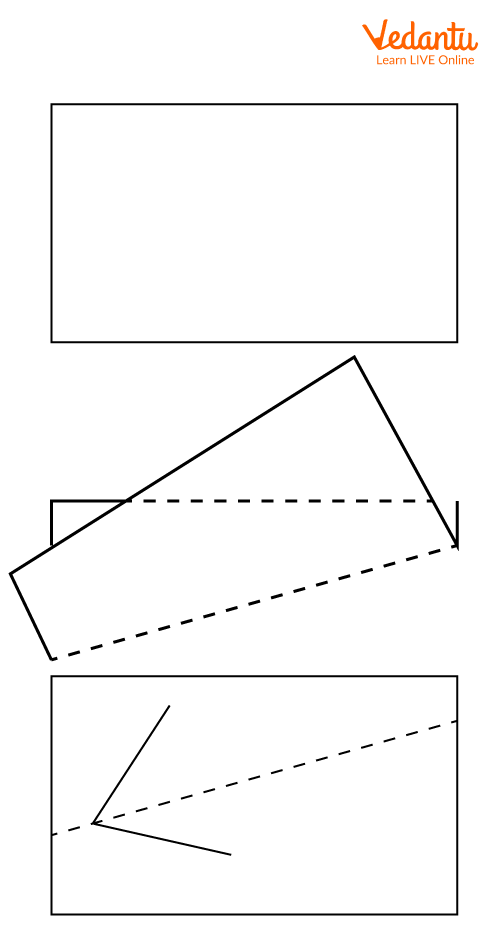Angle Bisector Using Paper Fold

## What are the Properties of Angle Bisectors?

It is essential to know the properties of angle bisectors. Since we cannot call all lines angle bisectors, we need to know a few distinct properties of angle bisectors. These are:

• It must pass through the vertex of the angle.

• It must be equally inclined[spaced] between the rays of the angle.

• The angle bisector is also the line of symmetry of the angle.

• In the case of a straight line, the angle bisector is perpendicular to the line.

For example, the first image shows a slice of pizza unevenly portioned by the line. Thus the line is not the angle bisector. However, the pizza is evenly portioned by the line in the second image. Hence it can be called an angle bisector.Pizza Showing Angle Bisector

## Construction of Angle Bisectors

Construction of Angle Bisectors using Protractor

We have to bisect ∠PQR. We measure the angle using a protractor. Let’s say the measure is 110°. The half of 110° is 55°. So we make an angle of measure 55°, called ∠PQS. QS is the required angle bisector. And ∠SQR $=$ ∠PQS $=$ 55°.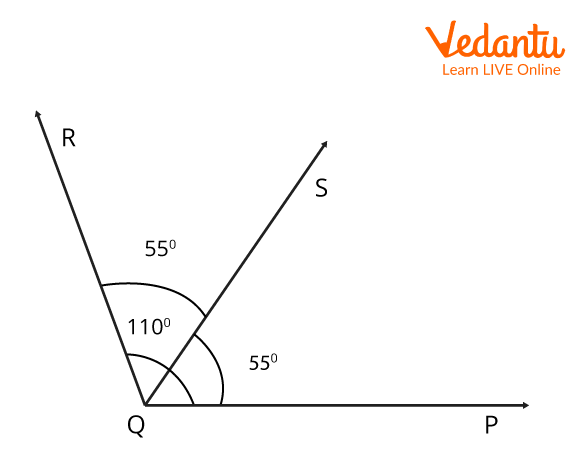Drawing Angle Bisector using a Protractor

Let us now move on to learn how to construct an angle bisector using a compass.

Construction of Angle Bisectors using a compass

We have to bisect ∠ABC. To do that,

• We first place the needle end of the compass on vertex B.

• Draw an arc, letting the arc intersect AB and BC at R and P, respectively.

• Now we place the needle end of the compass on P and draw a big enough arc.

• Do the same at point R and let these two arcs meet at point S.

• Join BS.

• We have our required angle bisector for ∠ABC.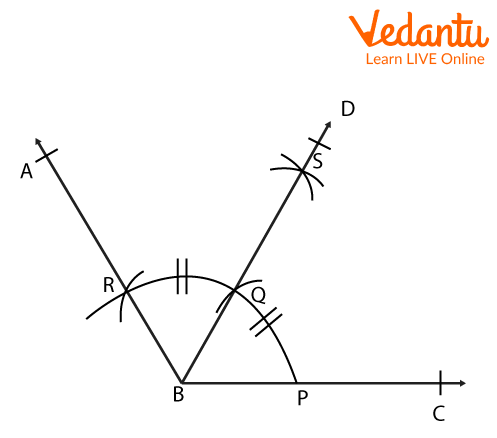Constructing Angle Bisector using Compass.

Great! You have now understood what angle bisectors are, their main properties, and their construction. Now, let us take a look at some solved examples below.

## Solved Examples

1. Given an ∠ABC $=$ 40°. Find the measure of the angle after an angle bisector bisects it.

Using the property that the angle bisector divides the angle into two equal parts, angle bisectors, we can find the measure of the angle. Let this measure be x. We have ${\rm{x + x}} = {40^ \circ }$. This gives ${\rm{x}} = {20^ \circ }$. Hence, the measure of the angle after its bisection is 20°.

2. Consider an angle ∠AOB. Write the steps to construct its angle bisector using a compass.

Steps to construct an angle bisector using a compass. First, draw the angle using a protractor.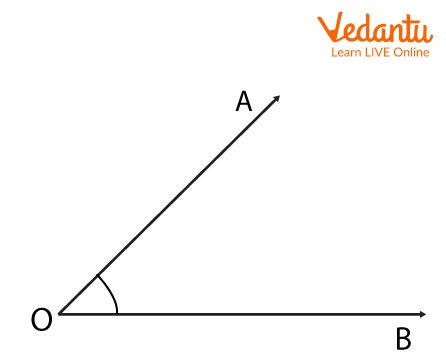Steps to Construct an Angle Bisector using a Compass

1. Make an arc of any radius using the compass with the needle on point O. Let’s name the points where the arc cuts the rays of angle AOB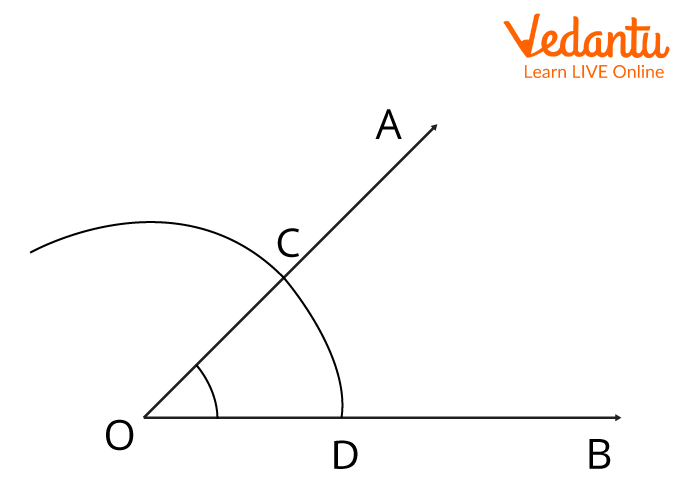Steps to Construct an Angle Bisector using a Compass

2. Now with the needle on Point C, draw an arc with a greater radius. Now put the needle on Point D and draw an arc of the same length. And let these arcs intersect at Point E.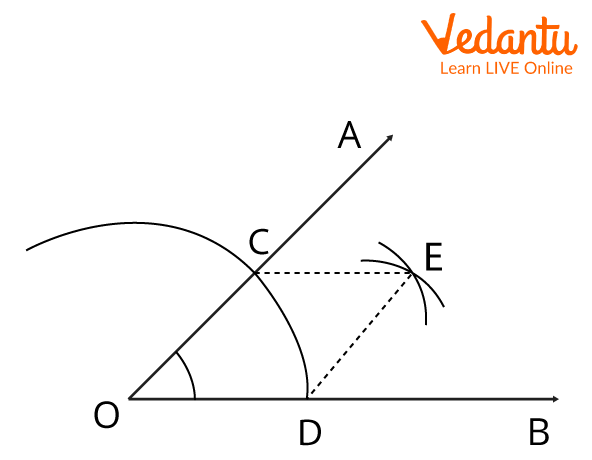Steps to Construct an Angle Bisector using a Compass

3. Join OE. This completes the construction of the angle bisector.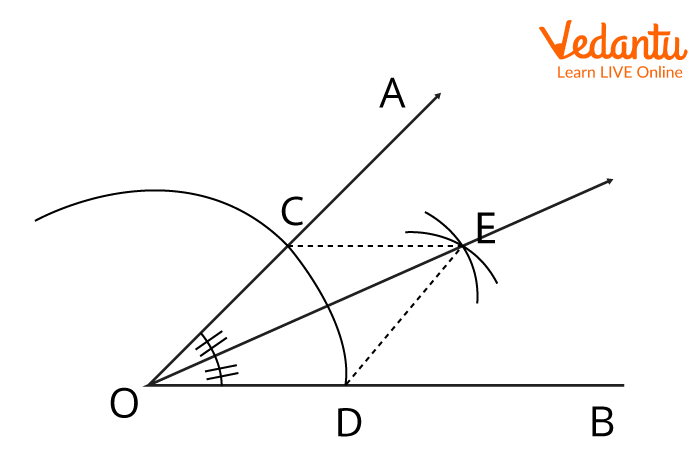Steps to Construct an Angle Bisector using a Compass

## Conclusion

The angle bisectors are very important in proving complicated proofs. Great! We now know how to identify angles, how to construct angle bisectors, and their construction and mathematical implementation of angle bisectors.

Last updated date: 28th Sep 2023
Total views: 70.8k
Views today: 0.70k

## FAQs on Understanding Angle Bisectors

1. How many angle bisectors does an angle have?

An angle has a unique angle bisector. However if we also choose to include the external angle, then there are precisely two angle bisectors for any angle formed by a pair of lines ( an internal angle bisector, and an external angle bisector).

2. Give one example of an angle in real life.

Take a book. Now open the cover page but keep it inclined at a position. What do you see? An angle formed between the book's page and the book's cover page.

3. What is the importance of angle bisectors?

Angle bisectors are very important in identifying the corresponding similar or identical parts of a triangle and many such polygons.

4. What is the range of value of a bisected angle?

The value of an angle ranges from 0° to 180°. Hence the value of the bisected angle ranges from 0° to 90°.